## research papersBIOLOGICALCRYSTALLOGRAPHY
ISSN: 1399-0047
Volume 62| Part 1| January 2006| Pages 58-64

## A qu­antitative approach to data-collection strategies

aMPG–ASMB c/o DESY, Notkestrasse 85b, 22607 Hamburg, Germany,bI. V. Shubnikov Institute of Crystallography, Russian Academy of Sciences, Leniski Prospect 59, Moscow, Russia, and cEMBL Hamburg Outstation, c/o DESY, Notkestrasse 85b, 22607 Hamburg, Germany
*Correspondence e-mail: sasha@embl-hamburg.de

(Received 1 June 2005; accepted 20 October 2005)

Statistical descriptors of the X-ray diffraction data set for a macromolecular crystal can be modelled using the information present in the initial diffraction images. Quantitative relationships between the crystal quality, beam apertures, oscillation width, resolution limit, redundancy and the data statistics are presented. They are analysed in terms of the radiation-dose requirements based on modelling in program the BEST. The influence of radiation damage on the data statistics is discussed.

### 1. Introduction

The main purpose of a crystallographic data collection is to extract the required structural information from a crystal given finite available experiment time and the limited crystal lifetime in an X-ray beam. Incorrect choice of data-collection strategy can lead to failure of the experiment.

The selection of data-acquisition parameters in the case of protein crystals is always a compromise between many requirements (see, for example, Arndt & Wonacott, 1977; Dauter, 1999; Pflugrath, 1999; Evans, 1999). Each approach has its advantages and disadvantages in terms of experimental constraints and goals (Borek et al., 2003). In this paper, we discuss the influence of data-acquisition parameters on the data quality using quantitative estimations of the relationships between these parameters and data-collection statistics.

### 2. Quantitative approach to the planning of data collection

Most of the parameters involved in data collection have to be considered individually for each experiment. There are two main geometrical parameters: the smallest total rotation range that provides a data set of desired completeness and the maximum oscillation step per frame that excludes reflection overlaps. These can be straightforwardly determined provided that the point group, unit-cell parameters and mosaicity are known (e.g. Ravelli et al., 1997; Leslie, 1992; Otwinowski & Minor, 2001). The choice of other parameters, e.g. the highest resolution of the data, optimum rotation width and scan speed, is more complicated owing to their complex relationships to the quality of a data set.

Recently, we reported a quantitative method for optimum data-collection planning employed in the software program BEST (Popov & Bourenkov, 2003).

The method is based on modelling the statistical characteristics of the data yet to be collected using the information derived from a few initial images taken with short exposure times. The modelling is based on well known features of protein crystal diffraction. Since the main uncertainties in the observed intensities are defined by counting statistics, they can be estimated using known diffraction and background intensities. For a majority of protein crystals, the probability density functions for diffraction intensities derived by Wilson (1949) are applicable. The expectation value of reflection intensity can be determined using a limited number of integral intensities and an empirical pattern of average squared structure-factor magnitudes.

The quality of measured diffraction data is usually judged by merging statistics and the mean ratio of intensities to their estimated uncertainties, I/σ(I), in the resolution shells. BEST can estimate these descriptors prior to data collection. It can also carry out inverse calculations and determine the values of parameters corresponding to given statistics.

### 3. Materials

Crystals of oxoanion polyreductase (OP) from Thioalkalivibrio nitratireducens were used for test-data collection and modelling. The crystals belong to the cubic space group, exhibit high diffraction quality (Table 1) and have an equi-dimensional rhombic dodecahedral habit. The measurements were carried out at beamlines BW6 (DESY) and ID29 (ESRF). The programs DENZO and SCALEPACK (Otwinowski & Minor, 1997) were used for data reduction and scaling.

 Table 1Crystal and data-collection parameters
 Space group P213 Unit-cell parameter (Å) 196 Overall ADP† (Å2) 15 Mosaicity (°) 0.25 Beamline BW6 ID29 Detector MAR CCD ADSC Q4 Wavelength (Å) 1.050 0.976
†Atomic displacement parameter.

### 4. Background scattering

The methods implemented in BEST assume that counting statistics are the major factor affecting the overall data statistics. Here, we demonstrate that they are mostly governed by the background (rather than the peak) counting statistics. Fig. 1shows partial reflection intensities of OP crystals collected to a resolution of 1.6 Å on BW6. Average background intensity (integrated over the peak area, 36 pixels, constant over the detector area) is also shown. Most of the reflection intensities are far below the background level in the resolution shell 3–1.6 Å, with a very few exceeding the background level at low resolution. The data statistics for a series of 84 such images are shown in Table 2and represent rather typical statistics in the data used for protein structure refinement.

 Table 2Data-processing statistics
 Values in parentheses are for the highest resolution shell.
 Resolution (Å) 12.0–1.6 (1.63–1.60) Completeness (%) 98.1 Redundancy 2.6 I/σ(I) 16.8 (2.0) R value (%) 6.3 (51.1)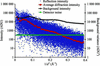Figure 1 25 500 partial reflection intensities and average integrated background scattering in the diffraction image of an OP crystal [0.25° rotation, 15 s exposure time (∼1012 photons), 0.25 × 0.25 × 0.25 mm crystal size, 0.3 × 0.3 mm slit size] as a function of resolution. The background variance component arising from detector noise is also shown.

In practice, the signal-to-noise ratio in the final data will also depend on the integration algorithms in the data-processing software. The counting statistic of the background intensity sets the lower limit of data accuracy, which cannot be reduced by means of data processing. Modern integration methods (Leslie, 2001; Kabsch, 2001; Pflugrath, 1999; Otwinowski & Minor, 2001) are sufficiently powerful to attain data accuracy very close to that defined by the counting statistics and instrumental factors under a broad variety of conditions. Inflation of the estimated standard deviation as a function of the intensity is essential for the strongest reflections, but has only a minor effect on the weak high-resolution data. This allows us to reduce the analysis to statistical considerations only.

Being a critical factor, the background distribution is worth a thorough analysis. In Fig. 2, the density of background components (intensity per detector pixel per second), i.e. scattering by the crystal embedded in a cryosolution (crystal probe), scattering by the cryosolution alone and scattering by the exogenous sources (air, slits, backstop etc.), are shown (data were measured on BW6, beam size 0.3 × 0.3 mm). Exogenous background scattering (excluding a probe) was proportional to the beam cross-section within a few percent (data not shown). It contributes to the total background significantly at low scattering angles. The shapes of the scattering distributions measured from the solution alone and solution containing the crystal are almost identical (the solvent content of OP crystals is 77%). The background scattering distributions for two probes containing crystals with different volumes (0.25 × 0.25 × 0.25 and 0.12 × 0.12 × 0.12 mm) are proportional to the volume.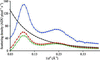Figure 2 Average background scattering density from OP crystals (blue and green curves) and from the loop with cryoliquid (red curve) corrected for scattering from beam stop and air (black curve) versus resolution. Beam was collimated by 0.3 × 0.3 mm slits. The blue and green lines correspond to crystals with dimensions 0.25 × 0.25 × 0.25 and 0.12 × 0.12 × 0.12 mm, respectively.

Another component of the background variance is introduced by detector-readout noise (see, for example, Pflugrath, 1999), which is independent of the X-ray scattering (e.g. of the exposure time). The r.m.s. deviation of an individual pixel value over an independent dark-current reading 〈σdark〉 of 2.6 ADU (analog-to-digital units) per pixel was measured for a MAR CCD. Given that the dark-current correction is determined by averaging two dark-current frames, the contribution of the detector noise to the total variance on a dark-current-corrected exposure is equal to 3/2〈σdark2 = 10 ADU2 per pixel. The straight line in Fig. 1shows this contribution.

### 5. Beam size

A change in the beam size alters the background scattering. The crystal diffraction intensity and the size and profile of the diffraction spots are affected when the beam is smaller than the crystal. To investigate the effect of the beam size on the data statistics, we carried out the following measurements on BW6. Diffraction images (five sequential images started at zero oscillation angle and five sequential images at 90°, all with 0.25° oscillation width and a maximum resolution of 1.6 Å) for each crystal have been measured using different beam apertures. The images were used for estimation of the total exposure time required to collect complete data with I/σ(I) = 3 in the outer resolution shell. Fig. 3shows the relative changes in the total exposure time as a function of the aperture.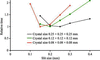Figure 3 Variation in exposure dose required to achieve I/σ(I) = 3 at resolution 1.6 Å versus slit size for three OP crystals. Curves are normalized to unity at their minima.

For a relatively large crystal (black line in Fig. 3) the shortest exposure time is achieved by setting the slit size close to the crystal size (about 0.25 mm). The use of the smaller slit size will require longer exposures owing to the reduction of diffraction intensity. An unreasonable increase in the aperture will cause an increase in the background and again require higher total dose of radiation. For a smaller 0.12 mm crystal, the 0.2 mm slit aperture provided the fastest data collection at this instrument. Beam collimation to 0.15 mm gives the best intensity-to-background ratio and hence the lowest total required dose, but reduces the flux density. Such a situation may be specific to a particular source and focusing conditions, but similar behaviour cannot be excluded, for instance, for focused beams at home X-ray generators.

An obvious rule of thumb for the choice of the apertures is that the beam should not be much larger then the size of the crystal. Owing to a variety of experimental features (crystal shape, thickness of the cryoliquid shell around the crystal, detector pixel size, variations in the beam profile and beam divergence as a function of the aperture), an accurate match may be difficult to attain from an optical view of the sample only. Empirical choice (comparing the total time/dose required for the data set) on the basis of a few diffraction images taken at different beam apertures would be more reliable. Setting the beam cross-section to significantly smaller then the sample size should be justified, e.g. by the requirements of spot separation on the detector. Comparing the slopes of the curves on the left and right sides of the minima (Fig. 3), one can see that the losses in diffracting volume are more expensive (in terms of compensating them by the exposure dose) compared with the increase in the background. The effect is more pronounced for high-resolution data, where the relative contribution of exogenous background scattering is lower.

### 6. Crystal

This section presents consideration of the required exposure dose as a function of the characteristics of the crystal: its size, overall atomic displacement parameter (ADP) and mosaicity. Here, we vary these parameters independently. Experimental background components (Fig. 2) and diffraction intensities from the crystal with size 0.25 mm have been used for modelling.

#### 6.1. Size

For simplicity, we assume that the crystal has a spherical shape and is surrounded by a cryoliquid shell of constant thickness. The diffraction spots on the detector were assumed to be circular with a diameter of six detector pixels. The change in the total dose relative to the 0.3 mm crystal for a 1.6 Å resolution data collection [I/σ(I) = 3] was calculated using BEST (Fig. 4a). It was assumed that the beam cross-section is equal to the probe cross-section, the diffraction intensities are proportional to the crystal volume, the background scattering is proportional to the total probe volume and exogenous scattering is proportional to the beam cross-section. For small crystals a dramatic increase in the exposure dose is required in order to compensate for the reduction in the signal-to-noise ratio. This dose increase is over an order of magnitude higher than that necessary to compensate for the decrease of the diffraction signal only. Even in the case of an ideally mounted crystal there would be a significant (approximately by a factor of five for a 0.02 mm crystal) difference arising from detector noise and air scattering.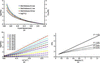Figure 4 (a) Relative change in the exposure dose required to achieve a resolution of 1.6 Å as a function of the crystal size and cryoliquid shell volume. The black curve shows variation in the crystal volume. (b) Relative change in the required exposure dose with respect to a crystal with an overall ADP B = 15 Å2 as a function of resolution. (c) Relative change in the required exposure dose for crystals with different mosaicity as a function of resolution. I/σ(I) = 3 in the outer resolution shell was used in all simulations.

Whatever the origin (thermal' or static') of atomic displacement in crystals is, the overall ADP is a measure of the exponential fall-off of the intensity with resolution. Theor­etically, the ADPs are related to the background scattering through the diffuse scattering term (e.g. Clarage & Phillips, 1997). In our experiments (see Fig. 2), the solution scattering and exogenous scattering essentially define the background level. Therefore, it can be considered to be independent of the ADPs. Fig. 4(b) shows the predicted relative change in radiation dose as a function of resolution for crystals with different ADPs. At the data-collection step, there are only limited possibilities to control this parameter, as well as the mosaicity: e.g. by screening, controlled dehydration (Kiefersauer et al., 2000), cryogenic annealing (e.g. Samygina et al., 2000) etc.

#### 6.3. Mosaicity

From the point of view of the data statistics, the strongest effect of the crystal mosaicity is a (linear) increase in the integration range of a diffraction reflection in φ (scan-axis coordinate). This increases the integrated background under the reflection and decreases the signal-to-noise ratio. Mosaicity is a factor that often limits the data resolution geometrically. For the resolution shells that are not affected by reflection overlaps, high mosaicity requires a higher exposure dose to achieve the same signal-to-noise ratio. The dependence of the required dose on the mosaicity is almost linear (Fig. 4c) and the slope depends on the ratio of the average diffraction intensity to the background scattering density. As a result, the required dose grows with mosaicity much faster at high resolution than at low resolution.

### 7. Slicing

In the past, the choice of the oscillation range (or rotation increment per image, Δφ) has been governed by practical considerations (e.g. managing large amounts of data and slow detector readout; Dauter & Wilson, 2001). Currently, there is a technical possibility of focusing on the minimization of the required radiation dose to achieve data of a given quality.

An appropriate choice of Δφ must be made to avoid spatial overlap of diffraction spots and to provide the minimal total dose of radiation to achieve the required data statistics. The maximum geometrically permitted oscillation range depends on the orientation of the crystal axes with respect to the beam. BEST takes this into account by applying the geometrical limit to relevant sub-ranges of the total rotation range only, while optimizing the exposure dose by varying Δφ within the geometrically permitted range.

In a perfect instrument, the dose required to obtain a certain signal-to-noise ratio would depend (approximately) linearly on the oscillation width (see Popov & Bourenkov, 2003). In practice, the total contribution of the detector-readout noise increases with the number of frames collected and thus defines an optimal Δφ.

In Fig. 5, the variation in the dose required to achieve I/σ(I) = 3 at resolutions of 1.6 and 2.0 Å for crystals with mosaicities of 0.25 and 0.75° is shown as a function of the oscillation width. The integral diffraction intensities and X-ray background scattering distributions were taken to be identical for all four simulations. The curves are normalized to the unity at the minima, i.e. to the dose corresponding to the optimal choice of Δφ. The shape of the curves is defined by the relative magnitudes of the diffraction signal, X-ray background and detector noise. It depends strongly on the mosaicity, although it would be difficult to define simple rules for choosing Δφ on the basis of the mosaicity alone. Generally, the costs of improper choice of Δφ are higher for lower values of mosaicity and weaker background scattering. Taking as an example the 1.6 Å data and 0.25° mosaicity, we estimated the Rmerge factors that would be obtained after a fixed total exposure dose (i.e. fixed scan speed) while varying Δφ. As shown in Fig. 6, both of the most frequent choices, 0.5 and 1.0° per frame, would notably degrade the data compared with the optimally chosen Δφ.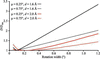Figure 5 Relative change in the required exposure dose (with respect to the dose Dmin corresponding to optimal Δφ) required to achieve I/σ(I) = 3 at resolution 1.6 and 2.0 Å versus oscillation width for crystals with mosaicity 0.25 and 0.75°.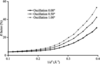Figure 6 Predicted Rmerge for data collections with the same rotation speed but different values of Δφ.

Mechanical errors affect fine-sliced data more than wide-sliced data (Pflugrath, 1999). On a good instrument the mechanical errors (e.g. shutter jitters) contribute only a few percent to the total variance. This contribution is important in an experiment aiming at highly accurate data [e.g. I/σ(I) > 20], but can be neglected in considerations of the high-resolution case with a typical signal-to-noise ratio of 2–3.

### 8. Data-collection resolution

The crystal-to-detector distance determines the geometrical resolution limit and spot separation on the diffraction image. The distance chosen must correspond to the exposure time (within the time/dose constraints), rotation range (redundancy) and Δφ.

The scattering component of the background drops as the square of the distance, whereas the reflection spot size (at least at the synchrotrons) changes much more slowly (e.g. Dauter, 1999). Increasing the distance improves the signal-to-noise ratio. Taking a chance by collecting the data to higher resolution by simply moving the detector closer may substantially impair the data statistics and in fact make the resolution lower. In Fig. 7, the data resolution, defined (arbitrarily) as the resolution where I/σ(I) = 3, and Rmerge at 1.6 Å resolution are plotted against the geometrical resolution limit. All other data-collection parameters were kept constant at the values that deliver data to a resolution of 1.6 Å. An attempt to increase the geometrical resolution to 1.2 Å actually resulted in an Rmerge that was twice as high at 1.6 Å and effectively reduced the resolution to 1.74 Å.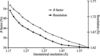Figure 7 Predicted resolution limit [as defined by I/σ(I) = 3] and Rmerge at 1.6 Å resolution versus geometrical resolution limit. Simulations were carried out assuming constant exposure time and oscillation width.

### 9. Total rotation range

The choice of the initial and final angles of the rotation range must assure the desired completeness of the data set. The smallest rotation range gives the data set with the minimum redundancy. Two perfect experiments with the same total exposure dose used for different rotation ranges would provide the same data statistics. However, in the presence of systematic error (resulting from, for example, integral non-linearity of the detector response, shutter jitters or crystal absorption), increasing the exposure dose per frame may not increase I/σ(I) for strong signals over a certain limit. In our model, the contribution of systematic error to the total intensity error equals 3% of intensity, i.e. I/σ(I) ≤ 33 for a single observation. Independent redundant measurements can improve the data statistics above this limit. On the other hand, increasing the number of frames by collecting redundant data results in stronger effects of the readout noise on weak signals.

For our test system, we calculated the dependence of I/σ(I) on resolution for two experiments using an equal total dose, one for a shortest rotation range equal to 21° and redundancy of 2.6 and the other for a full circle rotation (redundancy = 44) (Fig. 8). In both experiments, the exposure times per frame were sufficiently long to neglect jitters. The total dose was set to provide I/σ(I) = 3 in the outer shell in a full-circle experiment. A noticeable increase in I/σ(I) above 100 is achieved owing to the high redundancy in low-resolution shells < 3 Å, where the diffraction signals are extremely strong. The effect has already disappeared at 2 Å resolution. At 1.6 Å, the signal to noise is significantly impaired in a full-circle experiment. Owing to the reduced total contribution of the detector noise, the shortest rotation range gives I/σ(I) = 3 at 1.6 Å resolution at 40% lower dose compared with the full-circle experiment. As a general recommendation, high redundancy is necessary for measuring weak anomalous signals (∼1%) on well diffracting crystals (Usón et al., 2003). Straightforward estimation with BEST can provide useful information, e.g. the resolution at which the signal is sufficiently strong to be further improved by an increase in the redundancy. For data collection to the highest possible resolution (e.g. for accurate structure refinement) the shortest rotation range would normally be optimal.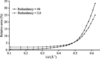Figure 8 Predicted average relative error [σ(I)/I] versus resolution for data collections with the same total radiation dose and different data redundancies.

Radiation damage occurs at all temperatures and leads to a resolution-dependent reduction in diffraction intensity and changes in the unit-cell parameters and crystal mosaicity as well as specific chemical modifications in the structure as a function of the absorbed radiation dose (reviewed by Ravelli & McSweeney, 2000). Although radiation-induced non-isomorphism and structural alterations at heavy-atom sites are often a limiting factor in phasing experiments, for high-resolution data collection [low I/σ(I) at the resolution limit] the major factor affecting data statistics is the loss of diffraction intensity. In Fig. 9the decay in diffraction intensity in four resolution shells is shown as a function of cumulative exposure. The whole crystal volume was exposed. The curves are recalculated from the results of standard frame-to-frame scaling. For modelling purposes, the curves were further smoothed by (log-) polynomial interpolation, extrapolated to longer exposures and used in BEST to estimate the signal-to-noise ratio in corresponding resolution shells as a function of exposure time (Fig. 10).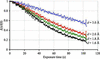Figure 9 Relative diffraction intensity, I(t)/I(0) = scale(t)exp[−B(t)sin2θ/λ2], of OP crystals versus exposure time accumulated at ESRF ID29 and resolution.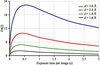Figure 10 Predicted I/σ(I) for a complete data set (200 frames, 0.1° oscillations) as a function of the exposure time per frame and resolution. Radiation damage as shown in Fig. 9.

In the hypothetical case when radiation damage is absent, the data statistics always improve with an increase in the exposure dose. The radiation damage drastically changes this behaviour. The statistics starts to degrade after reaching some limiting total exposure dose. The limiting total dose is lower for higher resolution than for low resolution. This fact should be taken into account for optimal experiment planning. One of the possible ways to determine these limits for a particular crystal type at a particular instrument is a preliminary experiment. The RADDOSE approach (Murray et al., 2005) combined with careful instrument calibration may provide a way to generalize the method in future.

### 11. Conclusions

A variety of different tasks, crystal characteristics and specific instrument conditions make it impossible to define rigid protocols for data collection that would be applicable in all cases. The appropriate decision has to be a result of a compromise between several competing requirements. Computationally efficient modelling of the data statistics for any combination of data-collection parameters provides a foundation for making the rational choice. In many cases this can be achieved in a fully automatic manner.

Crystal quality and radiation damage are the most important limitations for macromolecular crystallography. Crystal screening is required in order to find a crystal giving the required structural information. The modelling of data statistics using a few test images allows one to answer the question which crystal gives highest resolution with minimal radiation dose. Given a set of pre-tested isomorphous crystals, a more complicated plan of data collection can be constructed.

Modelling can also be used for empirical determination of optimal X-ray beam parameters such as aperture and divergence. Empirical determination of the optimal aperture can essentially improve data quality. Deviations from the optimum towards bigger and especially towards lower apertures requires increased exposure doses.

The choice of the total rotation range and rotation range per frame is not a purely geometrical problem. A proper choice of Δφ on the basis of data-statistics considerations permits substantial improvement of the high-resolution data statistics. Shortest rotation range provides minimum radiation dose in high-resolution data collection, whereas high data redundancy is necessary for collecting very accurate (SAD) data from strongly diffracting crystals.

The data resolution is uniquely defined for a given sample by the signal-to-noise requirements, experimental time and exposure dose constraints. Unjustified attempts to violate the limitations will result in poor data quality.

A careful strategy has to be used to extract the maximum amount of information taking into account the radiation damage. Provided that the intensity decay as a function of the dose is known, an objective resolution-dependent maximum of the total dose providing best data statistics can be found. This is of particular importance for MAD experiments, where high-accuracy data are required but the crystals are exposed to higher doses.

### Acknowledgements

We would like to thank V. Lamzin for useful discussions and help, K. Boiko and K. Poljakov for providing oxoanion polyreductase crystals and the ESRF staff for help at the beamline. The work was supported by the EC-funded project BIOXHIT (http://www.bioxhit.org ) contract No. LHSG-CT-2003-503420.

### ReferencesBIOLOGICALCRYSTALLOGRAPHY